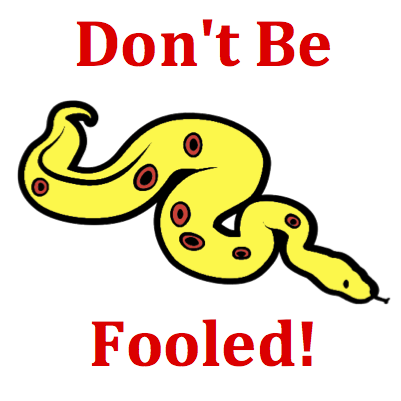# Vectors and Projectiles - Mission VP1 Detailed HelpThe diagram below shows three vectors at various orientations. According to the counterclockwise convention, the direction of the vector A (or B or C) is closest to ...Counterclockwise from East Convention for Vector Direction The direction of a vector is often expressed using the counterclockwise (CCW) convention. According to this convention, the direction of a vector is the number of degrees of rotation that the vector makes counterclockwise from East.Place the protractor such that the origin is located at the tail of the vector. Then measure the number of degrees that the vector makes with the East direction. The protractor is marked every 15 degrees. Thus, count by 15 degrees as you start at East and move counterclockwise around to the location of the vector.Many students become confused by the presence of the many vectors in this diagram. They are tempted to measure the angle between the indicated vector and another vector in the diagram. But don't be fooled! The presence of another vector does not affect the direction of the indicated vector. After all, if a vector points East, then it points East. The presence of another vector pointing southwest will not change the direction of a vector that points East. To determine the direction of a vector, ignore all other vectors and follow the strategy in the Game Plan section.